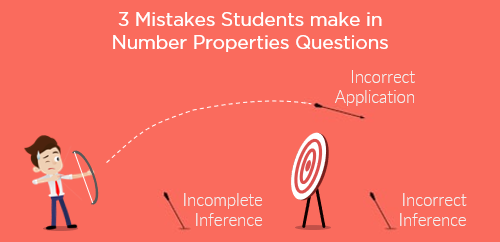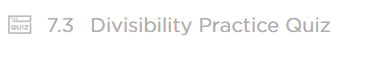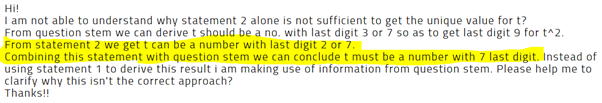GMAT Question of the Day - Daily to your Mailbox; hard ones only

 It is currently 15 Oct 2019, 02:02GMAT Club Daily Prep

Thank you for using the timer - this advanced tool can estimate your performance and suggest more practice questions. We have subscribed you to Daily Prep Questions via email.

Customized
for You

we will pick new questions that match your level based on your Timer History

Track

every week, we’ll send you an estimated GMAT score based on your performance

Practice
Pays

we will pick new questions that match your level based on your Timer History

Not interested in getting valuable practice questions and articles delivered to your email? No problem, unsubscribe here.GMAT Quant DS - 3 Mistakes Students Make in Number Properties Qs (II)

 new topic post reply Question banks Downloads My Bookmarks Reviews Important topics
Author Message
TAGS:

Hide Tags

e-GMAT RepresentativeV
Joined: 04 Jan 2015
Posts: 3074
GMAT Quant DS - 3 Mistakes Students Make in Number Properties Qs (II)  [#permalink]

Show Tags

GMAT Quant DS - 3 Mistakes Students Make in Number Properties Questions - Part IIThis is the second article in the series of 3 most common mistakes students make in the Number Properties DS question.
To read the first article: GMAT Quant DS - 3 Mistakes Students Make in Number Properties Questions - Part I

Highlights of the Previous Article

In the previous, we discussed why students make two of the three most common mistakes while solving a DS question.
These two mistakes were:
1. Drawing incorrect inference from the question stem.
2. Drawing incomplete inference from the question stem.
With the help of examples, we also learned how we can avoid these two mistakes.
Before reading further, we suggest that you read the first article if you have not read it yet.

In this article, we will discuss the third mistake that students make in a DS question.
• The third mistake is: Faltering while applying the inferences
We will also discuss how you can avoid doing this.

Mistake 3: Faltering while Applying the Inferences

In the first article, we learned how to avoid drawing incorrect and incomplete inferences.
• And, once we have mastered the skill of drawing correct and complete inferences, the next step is to apply those inferences correctly.

If this step is also executed properly, then you are 80% close to marking the correct answer choice.
• Why only 80%?
o Because reaming 20% is the application of the concepts.

So, let us look at one e-GMAT question to understand the 3rd most common type of mistake.

Question 1

If t is a positive integer, can $$t^2 + 1$$ be completely divided by 10?
1. $$91^2 * t$$ leaves a remainder of 1 when divided by 2.
2. $$91^2 * t = 5q + 2$$, where q is a non-negative integer.

(For e-GMAT students: This question is present in Divisibility practice quiz of Number properties module)Accuracy of this question is 41.85%
Total relevant forum post queries posted for this question: 13

• 58% of the students got this question wrong and out of this 58%, 60% of the students got this one wrong despite knowing the required concepts.

So, let us understand the question first.
Given:
• t is a positive integer.

To find:
• Whether $$t^2 + 1$$ is completely divisible by 10 or not.

Inference from what we need to find:
$$t^2 + 1$$ will be completely divisible by 10 when the last digit of $$t^2 + 1$$ is 0.
• That is, last digit of $$t^2$$ must be 9.
• And, it is only possible when last digit of t is either 3 or 7.

Hence, if last digit of t is either 3, or 7, then:
• Our answer will be yes.

If last digit of t is neither 3 nor 7 then answer will be NO.

Let us look at statement 2 where student faltered while applying this inference.
Statement 2: $$91^2 × t = 5q + 2$$, where q is a non-negative integer.

Student’s Mistake – Faltering while Applying the Inferences
Notice the highlighted part of this query.Wrong Application of Inference
The student thinks that:
• From question stem, t should be a number with last digit 3 or 7.
• From statement 2, t should be a number with last digit 2 or 7.
o Now, on combining both the results, we can conclude that t must be a number with last digit 7.

Here, I want you to think and find the mistake before reading any further.

Correct Application of Inference
First, I want to ask, did we infer that last digit of t can be 3 or 7 only?
• No!!!

We inferred that IF the last digit of t is either 3 or 7, then the answer will be YES.
Please notice the word “IF”.
• We still have to find out the exact value of units digit of t.
o However, the student assumes that the last digit of t is either 3 or 7 which is incorrect.
o From statement 2, last digit of t comes out to be 2 and 7.
 Now, we can apply the inference.
• For last digit 2-> $$t^2 + 1$$ is not divisible by 10.
• For last digit 7-> $$t^2 + 1$$ is completely divisible by 10.
o Hence, statement 2 is not sufficient to find the answer.

So, even when you have completely inferred all the inferences, you still need to apply them properly in order to mark the right answer.

How to Avoid Incorrect Application of Inferences
1. One reason for making this type of mistake can be because you might have solved a similar type of question in the past. And, assuming that this is the same question, you don’t pay much attention to read the question carefully and you incorrectly solve the question.
a. This type of mistake can be avoided by treating every question you come across as a new question and keeping an open mind to solve the question.

2. Keep a check on whether the inferred information is from the information given in the question stem or from the information that we need to find.

1. In this article, we learned how to avoid the 3rd most common mistake that students make in solving DS questions related to number properties.
2. We understood how important it is to read a question carefully.
a. Always be mindful of the keywords like – IF, BUT.
b. These keywords can decide the conditions when we will apply the inference.

3. Finally, always read every question with an open mind.
a. Do not solve a question thinking that you have solved a similar type of question previously.

If you consciously apply all the learnings from both the articles, you will surely see your accuracy and score improving.

Want to read more articles like this?

If you liked this article, then I am sure that you want to read some more articles like this.

To read all our article go here:Must Read Articles and Practice Questions to score Q51

You will also get a lot of practice questions to learn from.

Happy learning.
_________________
InternB
Joined: 29 Aug 2016
Posts: 24
Re: GMAT Quant DS - 3 Mistakes Students Make in Number Properties Qs (II)  [#permalink]

Show TagsRe: GMAT Quant DS - 3 Mistakes Students Make in Number Properties Qs (II)   [#permalink] 08 Apr 2019, 22:37
Display posts from previous: Sort by

GMAT Quant DS - 3 Mistakes Students Make in Number Properties Qs (II)

 new topic post reply Question banks Downloads My Bookmarks Reviews Important topics

 Powered by phpBB © phpBB Group | Emoji artwork provided by EmojiOne